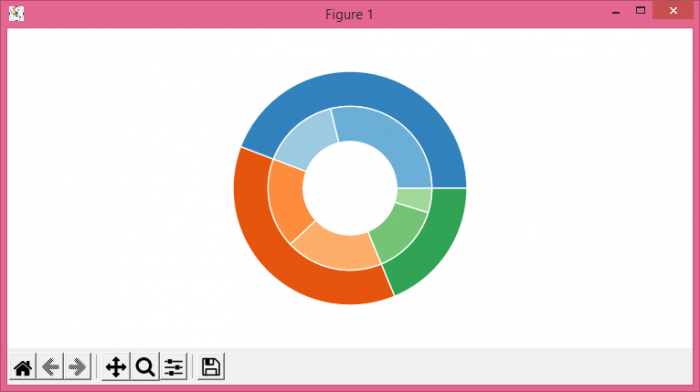# How to plot a nested pie chart in Matplotlib?

To plot a nested pie chart in Matplotlib, we can take the following steps −

• Set the figure size and adjust the padding between and around the subplots.
• Create a figure and a set of subplots.
• Initialize a variable size, create vals, cmap, outer_colors, inner_colors data using numpy.
• Use pie() function to make pie charts.
• To display the figure, use show() method.

## Example

import matplotlib.pyplot as plt
import numpy as np

plt.rcParams["figure.figsize"] = [7.50, 3.50]
plt.rcParams["figure.autolayout"] = True

fig, ax = plt.subplots()
size = 0.3
vals = np.array([[60., 32.], [37., 40.], [29., 10.]])
cmap = plt.get_cmap("tab20c")
outer_colors = cmap(np.arange(3)*4)
inner_colors = cmap([1, 2, 5, 6, 9, 10])

plt.show()